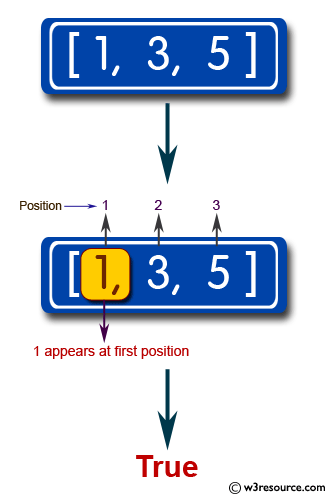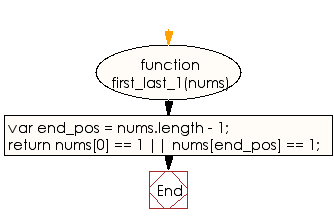# JavaScript: Check whether 1 appears in first or last position of a given array of integers

## JavaScript Basic: Exercise-71 with Solution

Write a JavaScript program to check whether 1 appears in first or last position of a given array of integers. The array length must be greater or equal to 1.

Pictorial Presentation:Sample Solution:

HTML Code:

``````<!DOCTYPE html>
<html>
<meta charset="utf-8">
<meta name="viewport" content="width=device-width">
<title>JavaScript program to check whether 1 appears in first or last position of a given array of integers. The array length must be greater or equal to 1.</title>
<body>

</body>
</html>
```
```

JavaScript Code:

``````function first_last_1(nums)
{
var end_pos = nums.length - 1;
return nums == 1 || nums[end_pos] == 1;
}

console.log(first_last_1([1, 3, 5]));
console.log(first_last_1([1, 3, 5, 1]));
console.log(first_last_1([2, 4, 6]));
```
```

Sample Output:

```true
true
false
```

Flowchart:ES6 Version:

``````function first_last_1(nums)
{
const end_pos = nums.length - 1;
return nums == 1 || nums[end_pos] == 1;
}

console.log(first_last_1([1, 3, 5]));
console.log(first_last_1([1, 3, 5, 1]));
console.log(first_last_1([2, 4, 6]));
``````

Live Demo:

See the Pen JavaScript - Check whether 1 appears in first or last position of a given array of integers - basic-ex-71 by w3resource (@w3resource) on CodePen.

What is the difficulty level of this exercise?

Test your Programming skills with w3resource's quiz.

﻿

## JavaScript: Tips of the Day

Checks if a string is an anagram of another string (case-insensitive, ignores spaces, punctuation and special characters)

Example:

```const isAnagram = (str1, str2) => {
const normalize = str =>
str
.toLowerCase()
.replace(/[^a-z0-9]/gi, '')
.split('')
.sort()
.join('');
return normalize(str1) === normalize(str2);
};
console.log(isAnagram('iceman', 'cinema')); // true
```

Output:

```true
```